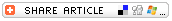#Explain Place ValuePlace value helps us determine the value of numbers. Our (base-10) number system contains numerals (or digits) only from 0 through 9, but we often need to use numbers greater than 9. We show numbers greater than 9 by using place value, which means that the value of a digit is determined by its place in the entire number. In the base-10 number system, each place has a value that's 10 times the value of the place immediately to its right.

• Numbers between zero and nine are represented by 1-digit numbers -- 8, for example. Numbers between ten and ninety-nine are represented by 2-digit numbers -- 58, for example. Numbers between one hundred and nine-hundred-ninety-nine are represented by 3-digit numbers -- 258, for example.
• Look at the number 258. The last digit on the right is in the ones' place. The number 258 contains 8 ones.
• The next digit to the left is in the tens' place. The value of a number in the tens' place is ten times the value of the same number in the ones' place. The number 258, therefore, contains 5 groups of ten.
• The next number to the left is in the hundreds' place. The value of a number in the hundreds' place is ten times the value of the same number in the tens' place. The number 258, therefore, contains 2 groups of one hundred.

## More Place Value Resources

Place Value Lesson Plans

Place Value Worksheets

Place Value Interactive Activities